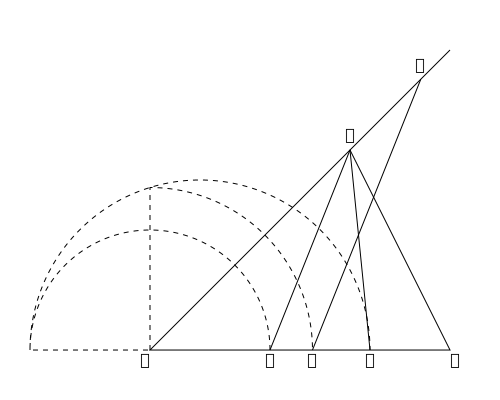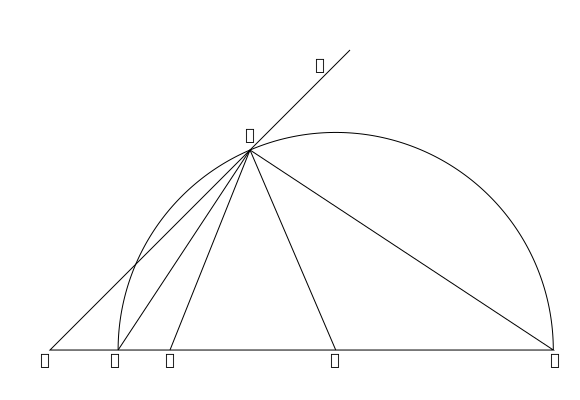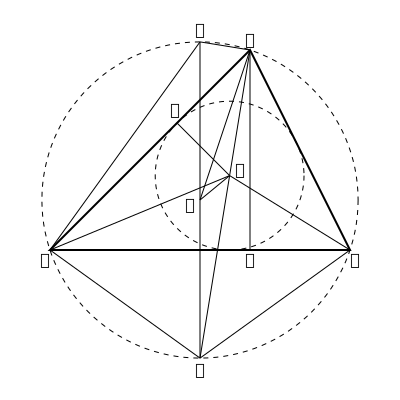output.to from Sideway
Draft for Information Only

# Content

`Elementary Geometry Miscellaneous Propositions Sources and References`

# Elementary Geometry

## Miscellaneous Propositions

931To divide the triangle 𝐴𝐵𝐶 in a given ratio by a line 𝑋𝑌 drawn parallel to any given line 𝐴𝐸.
Make 𝐵𝐷 to 𝐷𝐶 in the given ratio. Then make 𝐵𝑌 a mean proportional to 𝐵𝐸 and 𝐵𝐷, and draw 𝑌𝑋 parallel to 𝐸𝐴.
Proof: 𝐴𝐷 divides 𝐴𝐵𝐶 in the given ratio (VI. 1). Now 𝐴𝐵𝐸∶𝑋𝐵𝑌∷𝐵𝐸∶𝐵𝐷VI. 19 or ∷𝐴𝐵𝐸∶𝐴𝐵𝐷; therefore 𝑋𝐵𝑌=𝐴𝐵𝐷 932If the interior and exterior vertical angels at 𝑃 of the triangle 𝐴𝑃𝐵 be bisected by straight lines which cut the base in 𝐶 and 𝐷, then the circle circumscribing 𝐶𝑃𝐷 gives the locus of the vertices of all triangles on the base 𝐴𝐵 whose sides 𝐴𝑃, 𝐵𝑃 are in a constant ratio.
Proof: The ∠𝐶𝑃𝐷=12(𝐴𝑃𝐵+𝐵𝑃𝐸) =a right angle therefore 𝑃 lies on the circumference of the circle, diameter 𝐶𝐷 (III 31). Also 𝐴𝑃∶𝐵𝑃∷𝐴𝐶∶𝐶𝐵∷𝐴𝐷∶𝐷𝐵 (VI 3 and A.) a fixed ratio. 933 𝐴𝐷 is divided harmonically in 𝐵 and 𝐶; i.e. 𝐴𝐷∶𝐷𝐵∷𝐴𝐶∶𝐶𝐵; or the whole line is to one extreme part as the other extreme part is to the middle part. If we put 𝑎, 𝑏, 𝑐 of the lengths 𝐴𝐷, 𝐵𝐷, 𝐶𝐷, the proportion is expressed algebraically by 𝑎∶𝑏∷𝑎−𝑐∶𝑐−𝑏, which is equivalent to 1𝑎+1𝑏=2𝑐 934 Also 𝐴𝑃∶𝐵𝑃=𝑂𝐴∶𝑂𝐶=𝑂𝐶∶𝑂𝐵 and 𝐴𝑃2∶𝐵𝑃2=𝑂𝐴∶𝑂𝐵VI.19 𝐴𝑃2−𝐴𝐶2∶𝐶𝑃2∶𝐵𝑃2−𝐵𝐶2VI.3 & 𝐵 935If 𝑄 be the centre of the inscribed circle of the triangle 𝐴𝐵𝐶, and if 𝐴𝑄 produced meet the circumscribed circle, radius 𝑅, in 𝐹; and if 𝐹𝑂𝐺 be a diameter, and 𝐴𝐷 perpendicular to 𝐵𝐶; then 𝐹𝐶=𝐹𝑄=𝐹𝐵=2𝑅sin𝐴2i ∠𝐹𝐴𝐷=𝐹𝐴𝑂=𝐴2(𝐵−𝐶) and ∠𝐶𝐴𝐺=𝐴2(𝐵+𝐶)ii Proof of [i]: ∠𝐹𝑄𝐶=𝑄𝐶𝐴+𝑄𝐴𝐶 But 𝑄𝐴𝐶=𝑄𝐴𝐵=𝐵𝐶𝐹III.21 ∴𝐹𝑄𝐶=𝐹𝐶𝑄; ∴𝐹𝐶=𝐹𝑄; Similarly 𝐹𝐵=𝐹𝑄 Also ∠𝐺𝐶𝐹 is a right angle, and 𝐹𝐺𝐶=𝐹𝐴𝐶=12𝐴III.21 ∴𝐹𝐶=2𝑅sin𝐴2 936 If 𝑅, 𝑟 be the radii of the circumscribed and inscribed circles of the triangle 𝐴𝐵𝐶 (see last figure), and 𝑂, 𝑄 the centres; then 𝑂𝑄2=𝑅2−2𝑅𝑟 Proof: Draw 𝑄𝐻 perpendicular to 𝐴𝐶; then 𝑄𝐻=𝑟. By the isosceles triangle 𝐴𝑂𝐹, 𝑂𝑄2=𝑅2−𝐴𝑄⋅𝑄𝐹 (922, iii), and 𝑄𝐹=𝐹𝐶 (935,i), and by similar triangles 𝐺𝐹𝐶, 𝐴𝑄𝐻, 𝐴𝑄∶𝑄𝐻∷𝐺𝐹∶𝐹𝐶; therefore 𝐴𝑄⋅𝐹𝐶=𝐺𝐹⋅𝑄𝐻=2𝑅𝑟

## Sources and References

https://archive.org/details/synopsis-of-elementary-results-in-pure-and-applied-mathematics-pdfdrive

ID: 210900018 Last Updated: 9/18/2021 Revision: 0 Ref:References

1. Hilbert, D. (translated by Townsend E.J.), 1902, The Foundations of Geometry
2. Moore, E.H., 1902, On the projective axioms of geometry
3. Fitzpatrick R. (translated), Heiberg J.L. (Greek Text), Euclid (Author), 2008, Euclid's Elements of GeometryHome 5

Management

HBR 3

Information

Recreation

Culture

Chinese 1097

English 337

Computer

Hardware 156

Software

Application 207

Latex 35

Manim 203

Numeric 19

Programming

Web 285

Unicode 504

HTML 65

CSS 65SVG 9

ASP.NET 270OS 422

Python 66Knowledge

Mathematics

Algebra 84

Geometry 32

Calculus 67

Engineering

Mechanical

Rigid Bodies

Statics 92

Dynamics 37

Control

Natural Sciences

Electric 27